# tachorpm

Extract RPM signal from tachometer pulses

## Syntax

``rpm = tachorpm(x,fs)``
``````[rpm,t,tp] = tachorpm(x,fs)``````
``[___] = tachorpm(x,fs,Name,Value)``
``tachorpm(___)``

## Description

````rpm = tachorpm(x,fs)` extracts a rotational speed signal, `rpm`, from a tachometer pulse signal vector, `x`, that has been sampled at a rate of `fs` Hz.If you do not have a tachometer pulse signal, use `rpmtrack` to extract `rpm` from a vibration signal.```
``````[rpm,t,tp] = tachorpm(x,fs)``` also returns the time vector, `t`, and the detected pulse locations, `tp`.```
````[___] = tachorpm(x,fs,Name,Value)` specifies options using `Name,Value` pairs and any of the previous syntaxes.```

example

````tachorpm(___)` with no output arguments plots the generated RPM signal and the tachometer signal with the detected pulses.```

## Examples

collapse all

Load a simulated tachometer signal sampled at 300 Hz.

```load tacho ```

Compute and visualize the RPM signal using `tachorpm` with the default values.

```tachorpm(Yn,fs) ```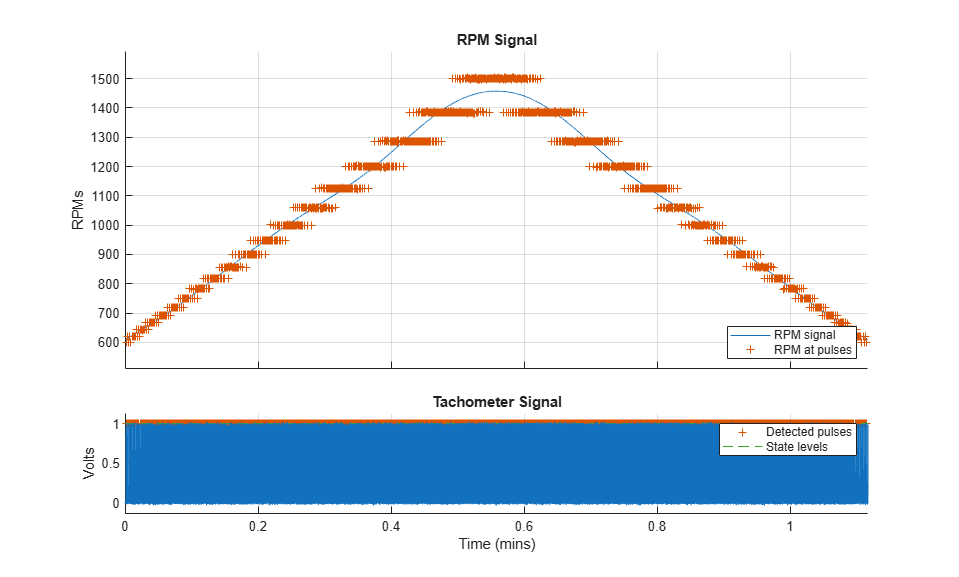Increase the number of fit points to capture the RPM peak. Too many points result in overfitting. Verify this result by zooming in on the area around the peak.

```tachorpm(Yn,fs,'FitPoints',600) axis([0.47 0.65 1320 1570]) ```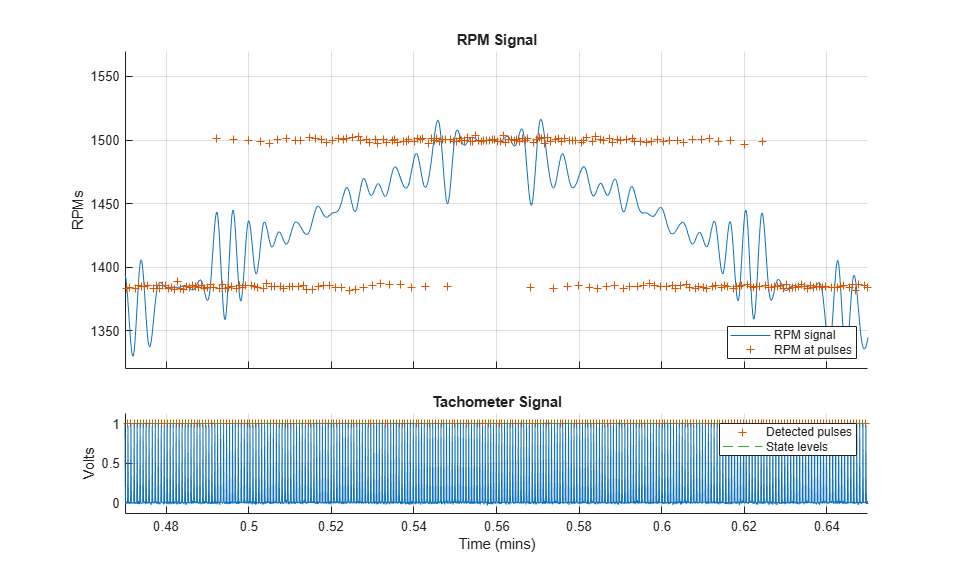Choose a moderate number of points to obtain a better result.

```tachorpm(Yn,fs,'FitPoints',100) ```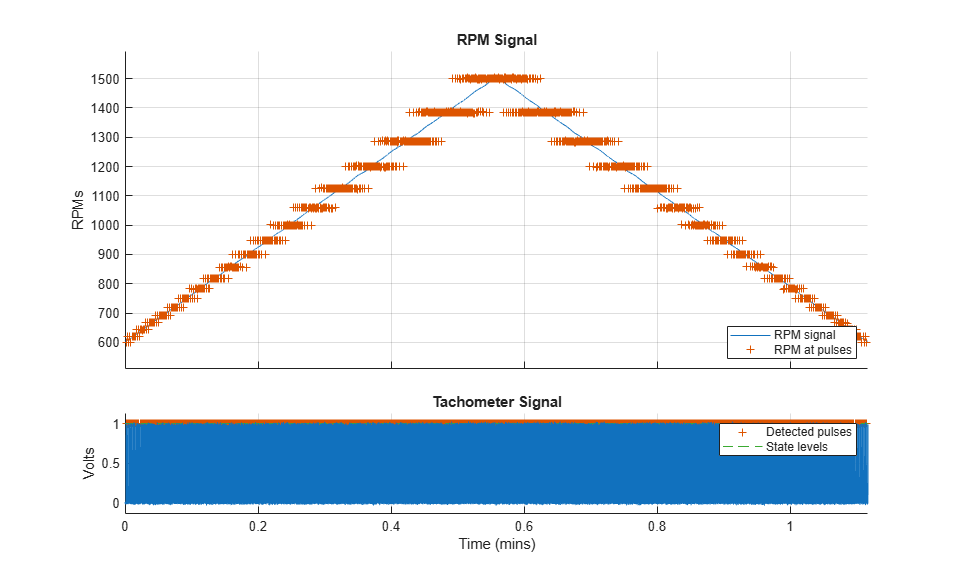Add white Gaussian noise to the tachometer signal. The default pulse-finding mechanism misses pulses and returns a jagged signal profile. Verify this result by zooming in on a two-second time interval.

```rng default wgn = randn(size(Yn))/10; Yn = Yn+wgn; [rpm,t,tp] = tachorpm(Yn,fs,'FitPoints',100); figure plot(t,Yn,tp,mean(interp1(t,Yn,tp))*ones(size(tp)),'+') hold on sl = statelevels(Yn); plot(t,sl(1)*ones(size(t)),t,sl(2)*ones(size(t))) hold off xlim([9 10]) ```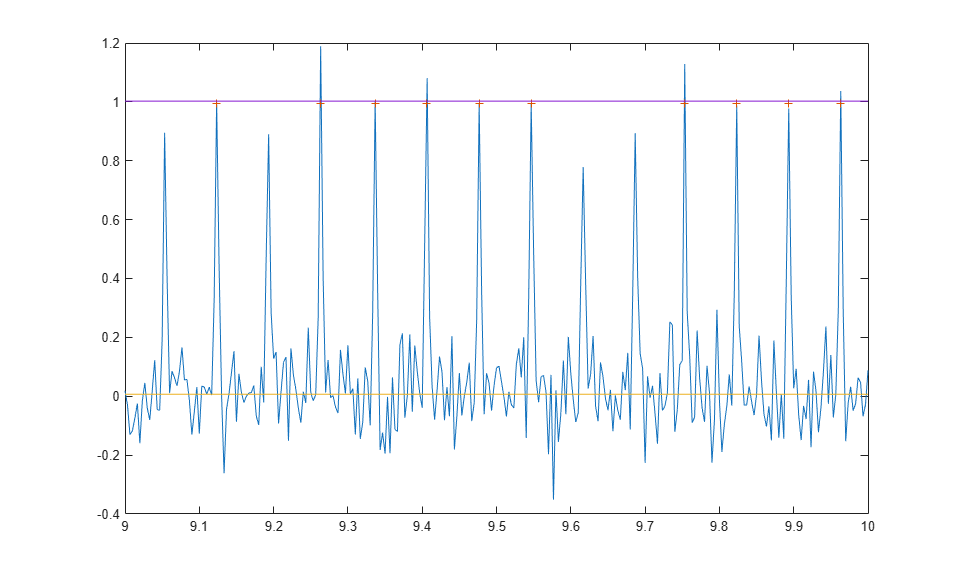Adjust the state levels to improve the pulse finding.

```sl = [0 0.75]; [rpm,t,tp] = tachorpm(Yn,fs,'FitPoints',100,'StateLevels',sl); plot(t,Yn,tp,mean(interp1(t,Yn,tp))*ones(size(tp)),'+') hold on plot(t,sl(1)*ones(size(t)),t,sl(2)*ones(size(t))) hold off xlim([9 10]) ```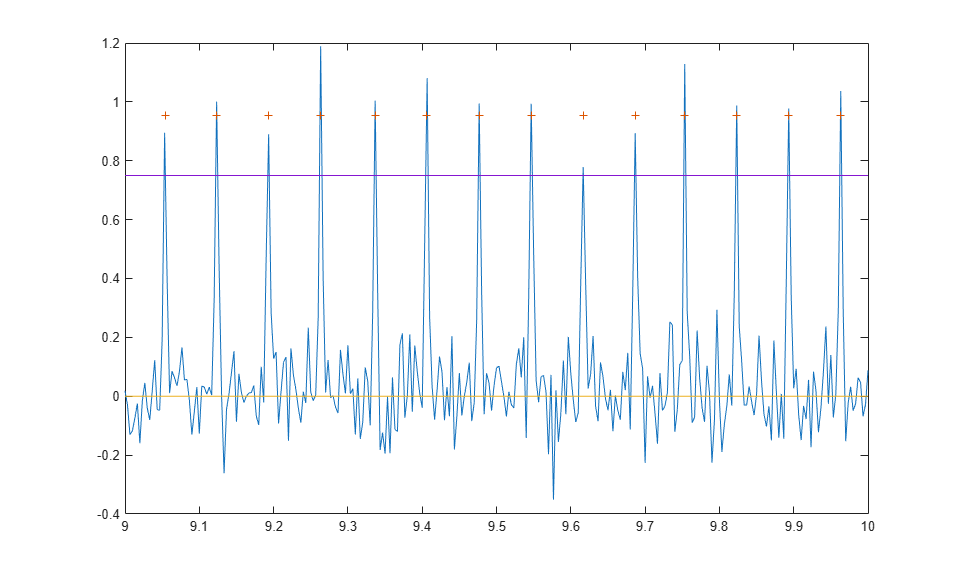## Input Arguments

collapse all

Tachometer pulse signal, specified as a row or column vector.

Example: `double(chirp((-1.5:1/2e2:1.5),14,1.1,8,'quadratic')>0.98)` resembles a tachometer signal, sampled for three seconds at 200 Hz, and obtained during a quadratic run-up/coast-down test.

Sample rate, specified as a positive scalar expressed in Hz.

### Name-Value Arguments

Specify optional comma-separated pairs of `Name,Value` arguments. `Name` is the argument name and `Value` is the corresponding value. `Name` must appear inside quotes. You can specify several name and value pair arguments in any order as `Name1,Value1,...,NameN,ValueN`.

Example: `'PulsesPerRev',3,'OutputFs',1e3` specifies that there are three tachometer pulses per revolution and that the returned RPM signal is to be sampled at 1 kHz.

Number of tachometer pulses per revolution, specified as the comma-separated pair consisting of `'PulsesPerRev'` and a real scalar.

State levels used to identify pulses, specified as the comma-separated pair consisting of `'StateLevels'` and a two-element real vector. The first element of the vector corresponds to the low-state level and the second element corresponds to the high-state level. Choose the state levels so that all pulse edges cross within 10% of both of them. If this option is not specified, then `tachorpm` computes the levels automatically using the histogram method, as in the `statelevels` function.

Output sample rate, specified as the comma-separated pair consisting of `'OutputFs'` and a real scalar.

Fitting method, specified as the comma-separated pair consisting of `'FitType'` and one of either `'smooth'` or `'linear'`.

• `'smooth'` — Fit a least-squares B-spline to the pulse RPM values.

• `'linear'` — Interpolate linearly between pulse RPM values.

B-spline breakpoints, specified as the comma-separated pair consisting of `'FitPoints'` and a real scalar. The number of breakpoints is a trade-off between curve smoothness and closeness to the underlying data. Choosing too many breakpoints can result in overfitting. This argument is ignored if `'FitType'` is set to `'linear'`.

## Output Arguments

collapse all

Rotational speeds, returned as a vector expressed in revolutions per minute. `rpm` has the same length as `x`.

Time vector, returned as a vector of positive values expressed in seconds.

Pulse locations, returned as a vector of positive values expressed in seconds.

## Algorithms

The `tachorpm` function performs these steps:

1. Uses `statelevels` to determine the low and high states of the tachometer signal.

2. Uses `risetime` and `falltime` to find the times at which each pulse starts and ends. It then averages these readings to locate the time of each pulse.

3. Uses `diff` to determine the time intervals between pulse centers and computes the RPM values at the interval midpoints using RPM = 60 / Δt.

4. If `'FitType'` is specified as `'smooth'`, then the function performs least-squares fitting using splines. If `'FitType'` is specified as `'linear'`, then the function performs linear interpolation using `interp1`.

 Brandt, Anders. Noise and Vibration Analysis: Signal Analysis and Experimental Procedures. Chichester, UK: John Wiley & Sons, 2011.

 Vold, Håvard, and Jan Leuridan. “High Resolution Order Tracking at Extreme Slew Rates Using Kalman Tracking Filters.” Shock and Vibration. Vol. 2, 1995, pp. 507–515.